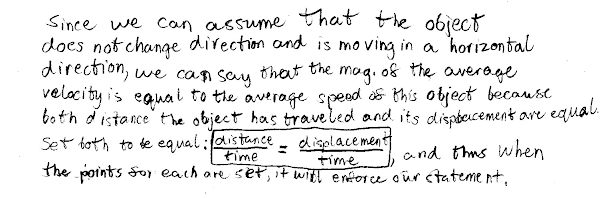## 20141101

### Physics midterm question: average velocity magnitude equal to average speed

Physics 205A Midterm 1, fall semester 2014
Cuesta College, San Luis Obispo, CA

Cf. Giambattista/Richardson/Richardson, Physics, 2/e, Problem 2.11, Conceptual Question 3.9The vx(t) graph of an object is shown at right. The object starts at x = 0 at t = 0. Discuss why the magnitude of the average velocity is equal to the average speed of this object, for the time interval 0 ≤ t ≤ 10 s. Explain your reasoning by using the properties of position, distance traveled, displacement, velocity, and speed.

• p:
Correct. Discusses/demonstrates:
1. Horizontal velocity is always positive (vx(t) graph does not dip below time axis), so the object is always traveling in the positive (forward) direction, and thus magnitude of displacement must be equal to distance traveled;
2. As magnitude of average velocity is the magnitude of displacement over time, and average speed is distance traveled over time, since magnitude of displacement is equal to distance traveled, then magnitude of average velocity is equal to the average speed.
• r:
As (p), but argument indirectly, weakly, or only by definition supports the statement to be proven, or has minor inconsistencies or loopholes. Typically does not explicitly support from the vx(t) graph why magnitude of displacement must be equal to distance traveled.
• t:
Nearly correct, but argument has conceptual errors, or is incomplete.
• v:
Limited relevant discussion of supporting evidence of at least some merit, but in an inconsistent or unclear manner.
• x:
Implementation of ideas, but credit given for effort rather than merit.
• y:
Irrelevant discussion/effectively blank.
• z:
Blank.
Sections 70854, 70855, 73320
Exam code: midterm01m0oU
p: 24 students
r: 38 students
t: 5 students
v: 5 students
x: 2 students
y: 0 students
z: 0 students

A sample "p" response (from student 0203):A sample "r" response (from student 4129):